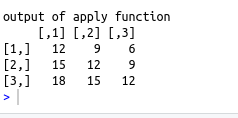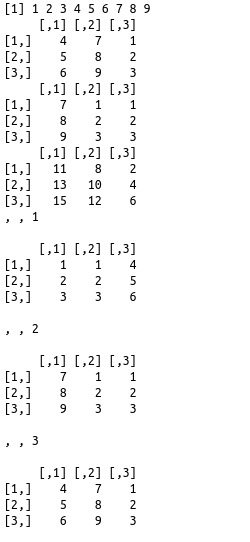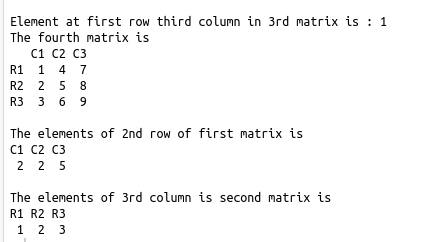• #5, First Floor, 4th Street Dr. Subbarayan Nagar Kodambakkam, Chennai-600 024 Landmark : Samiyar Madam
• pro@slogix.in
• +91- 81240 01111

### What is Arrays in R?

###### Description

To understand the arrays in R and its computations.

###### Process
• Arrays stores datas in more than two dimension.
• Creating an array of (3,2,5) creates 5 matrices each having 3 rows and 2 columns
• array(C(),dim,dimnames) is used to create an array

Syntax :

array(c(),dim,dimnames)

• c() – Vector input for the elements
• dim – parameter for the no.of rows columns and no.of matrices
• dimnames – this parameter is used to give names to rows and columns.The names are given as list

Accessing array elements :

The elements of the array can be accessed by their row number and column number as indexing value.

Example : a[,,3],a[1,,2],a[3,1,2],a[,2,1]

• a[,,3] – Gives the Third matrix
• a[1,,2] – first row of second matrix
• a[3,1,2] – third row first column of second matrix
• a[,2,1] – Second column of first matrix

Computing array elements :

• Computations on elements of array are carried out by accessing elements of the matrices.

Computation across array elements :

• Computations across elements can be done using the apply()
###### Sapmle Code

rownames=c(“R1″,”R2″,”R3”)
columnnames=c(“C1″,”C2″,”C3”)
v1=c(1:3)
v2=c(1:9)
a=array(c(v1,v2),dim=c(3,3,4),dimnames=list(rownames,columnnames))
cat(“The array a is\n”)
print(a)
cat(“\nElement at first row third column in 3rd matrix is :”,a[1,3,3])
cat(“\nThe fourth matrix is \n”)
print(a[,,4])
cat(“\nThe elements of 2nd row of first matrix is \n”)
print(a[2,,1])
cat(“\nThe elements of 3rd column is second matrix is \n”)
print(a[,3,2])

#Array computation and accessing elements of matrices
v1=c(1:3)
v2=c(1:9)
print(v2)
a=array(c(v1,v2),dim=c(3,3,3))

#dimnames=list(rownames,columnnames))
m1=a[,,3]
m2=a[,,2]
print(m1)
print(m2)
print(m1+m2)
print(a)
cat(“output of apply function\n”)
print(apply(a,c(1,2),sum))

###### Screenshots